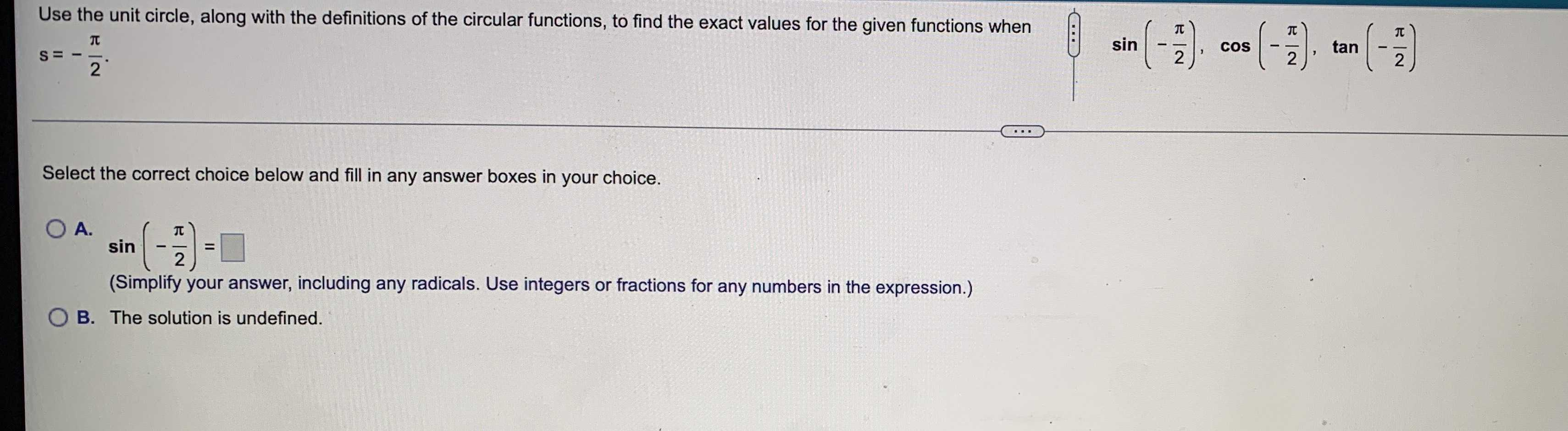### Still have math questions?

Trigonometry
QuestionUse the unit circle, along with the definitions of the circular functions, to find the exact values for the given functions when

$$s = - \frac { \pi } { 2 }$$ . Select the correct choice below and fill in any answer boxes in your choice. A. $$\sin ( - \frac { \pi } { 2 } ) =$$